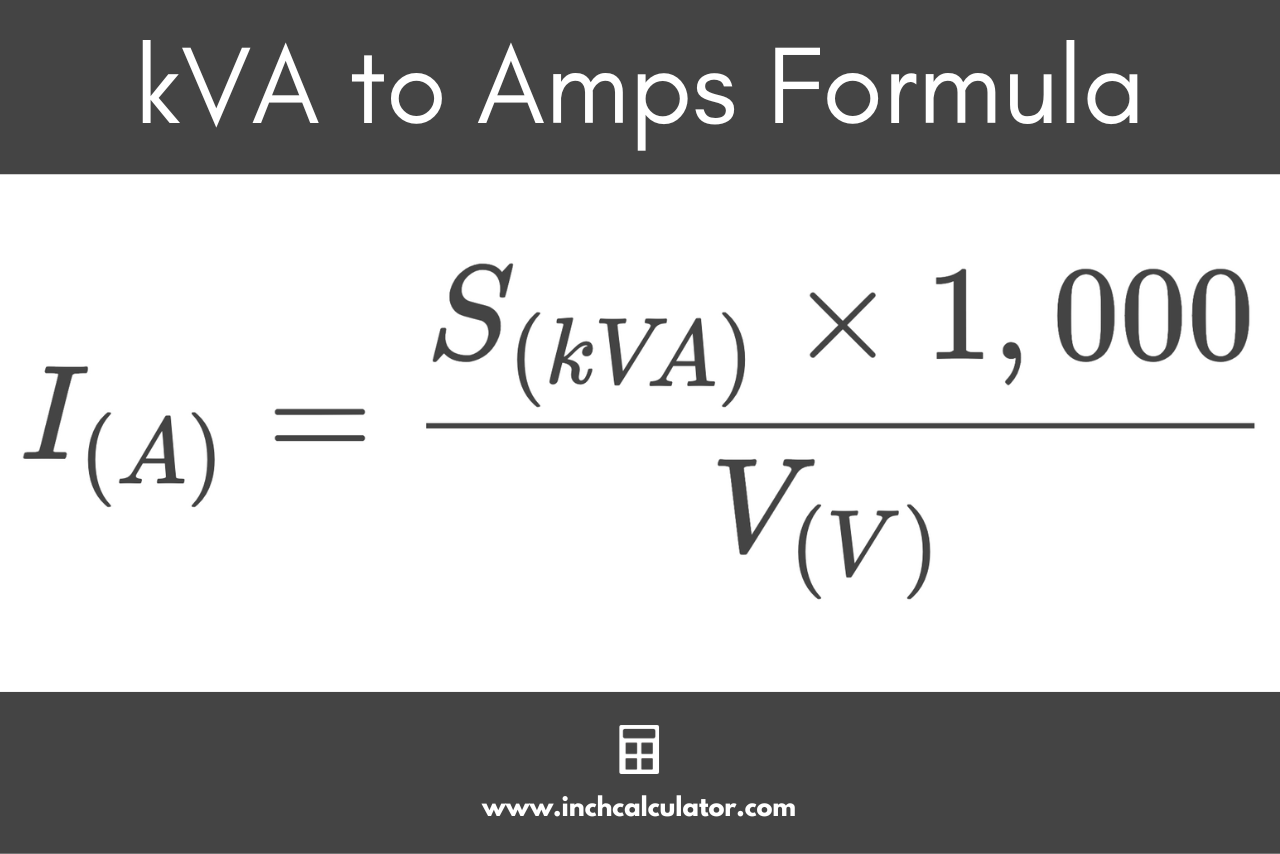# Kilovolt-Amps (kVA) to Amps Conversion Calculator

Convert kVA to amps (A) by entering the power in kVA and voltage below. Optionally, calculate for a three-phase electrical circuit by choosing the phase.

kVA
volts

## Current:

Amps
Learn how we calculated this below

## How to Convert kVA to Amps

kVA, short for kilovolt-amps, is a measure of the apparent power in an electrical circuit. One kVA is equal to 1,000 volt-amps and they are most commonly used for measuring apparent power in generators and transformers.

Amps are a measure of electrical current in a circuit.

In order to convert from kVA to amps, we’ll also need the RMS voltage of the circuit, and then we can use the formula for power

### Kilovolt-Amps to Amps Formula

The formula to convert kVA to amps is:

I(A) = S(kVA) × 1,000 / V(V)

This means that the current I in amps is equal to the apparent power S in kVA times 1,000, divided by the RMS voltage V.For example, let’s find the current for a 220-volt circuit with 25 kVA of apparent power.

I(A) = 25 kVA × 1,000 / 220 V
I(A) = 113.64 A

Do you want to convert amps to kVA?

## How to Convert kVA to Amps in Three-Phase Circuits

The formula to convert kVA to amps in a three-phase circuit looks like this:

I(A) = S(kVA)× 1,000 / √3 × VL-L(V)

For a balanced load where the current is the same on all phases, the current I in amps is equal to 1,000 times the apparent power S in kVA divided by the square root of 3 (1.732) times the line to line voltage V.

For example, let’s find the current for a 440-volt three-phase circuit with 50 kVA of apparent power.

I(A) = 50 kVA × 1,000 / 1.732 × 440 V
I(A) = 65.608 A

## kVA to Amps Conversion Chart

The table below shows kVA converted to amps at various voltages in a three-phase AC circuit.

Chart showing kilovolt-amps (kVA) converted to amps (A) at various voltages for a three-phase AC circuit.
Apparent Power in kVA Current in Amps at
208 V 220 V 240 V 440 V 480 V
1 kVA 2.78 A 2.62 A 2.41 A 1.31 A 1.2 A
2 kVA 5.55 A 5.25 A 4.81 A 2.62 A 2.41 A
3 kVA 8.33 A 7.87 A 7.22 A 3.94 A 3.61 A
4 kVA 11.1 A 10.5 A 9.62 A 5.25 A 4.81 A
5 kVA 13.88 A 13.12 A 12.03 A 6.56 A 6.01 A
6 kVA 16.65 A 15.75 A 14.43 A 7.87 A 7.22 A
7 kVA 19.43 A 18.37 A 16.84 A 9.19 A 8.42 A
8 kVA 22.21 A 20.99 A 19.25 A 10.5 A 9.62 A
9 kVA 24.98 A 23.62 A 21.65 A 11.81 A 10.83 A
10 kVA 27.76 A 26.24 A 24.06 A 13.12 A 12.03 A
25 kVA 69.39 A 65.61 A 60.14 A 32.8 A 30.07 A
50 kVA 138.8 A 131.2 A 120.3 A 65.61 A 60.14 A
75 kVA 208.2 A 196.8 A 180.4 A 98.41 A 90.21 A
100 kVA 277.6 A 262.4 A 240.6 A 131.2 A 120.3 A
200 kVA 555.1 A 524.9 A 481.1 A 262.4 A 240.6 A
300 kVA 832.7 A 787.3 A 721.7 A 393.6 A 360.8 A
400 kVA 1,110 A 1,050 A 962.3 A 524.9 A 481.1 A
500 kVA 1,388 A 1,312 A 1,203 A 656.1 A 601.4 A
600 kVA 1,665 A 1,575 A 1,443 A 787.3 A 721.7 A
700 kVA 1,943 A 1,837 A 1,684 A 918.5 A 842 A
800 kVA 2,221 A 2,099 A 1,925 A 1,050 A 962.3 A
900 kVA 2,498 A 2,362 A 2,165 A 1,181 A 1,083 A
1,000 kVA 2,776 A 2,624 A 2,406 A 1,312 A 1,203 A
2,000 kVA 5,551 A 5,249 A 4,811 A 2,624 A 2,406 A
3,000 kVA 8,327 A 7,873 A 7,217 A 3,936 A 3,608 A
4,000 kVA 11,103 A 10,497 A 9,623 A 5,249 A 4,811 A
5,000 kVA 13,879 A 13,122 A 12,028 A 6,561 A 6,014 A
6,000 kVA 16,654 A 15,746 A 14,434 A 7,873 A 7,217 A
7,000 kVA 19,430 A 18,370 A 16,839 A 9,185 A 8,420 A
8,000 kVA 22,206 A 20,995 A 19,245 A 10,497 A 9,623 A
9,000 kVA 24,982 A 23,619 A 21,651 A 11,809 A 10,825 A
10,000 kVA 27,757 A 26,243 A 24,056 A 13,122 A 12,028 A

You might also be interested in our volt-amps to amps or our kilowatts to amps calculators.

## References

1. Miller, C., NFPA's Electrical References, National Fire Protection Association, 2004, Jones & Bartlett Learning, 67-75. https://www.google.com/books/edition/NFPA_s_Electrical_References/raUyIi7i-asC
2. Miller, C., Ugly’s Electrical References, 2020 Edition, 2020, Jones & Bartlett Learning, 16-23. https://books.google.com/books?id=1kS8DwAAQBAJ